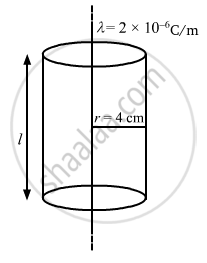Advertisement Remove all ads

# Find the Magnitude of the Electric Field at a Point 4 Cm Away from a Line Charge of Density 2 × 10 − 6 C M − 1 . - Physics

Answer in Brief

Find the magnitude of the electric field at a point 4 cm away from a line charge of density 2 × 10-6 Cm-1.

Advertisement Remove all ads

#### Solution

Given:
Charge density of the line containing charge, λ = 2 × 10-6 C/m-1

We need to find the electric field at a distance of 4 cm away from the line charge.
We take a Gaussian surface around the line charge of cylindrical shape of radius r = 4 ×10-2 m and height l.The charge enclosed by the Gaussian surface, qen = λl
Let the magnitude of the electric field at a distance of 4 cm away from the line charge be E.

Thus, net flux through the Gaussian surface, phi = oint  vec"E" . vec"d.s"
There will be no flux through the circular bases of the cylinder; there will be flux only from the curved surface.
The electric field lines are directed radially outward, from the line charge to the cylinder.
Therefore, the lines will be perpendicular to the curved surface.
Thus,

phi = oint  vec"E" . vec "d.s" = "E" oint   "ds"

=> phi = "E" xx 2 pi "r""l"

Applying Gauss's theorem,

phi = (q_"en")/∈_0

"E" xx 2 pi "r""l" =( λ"l")/∈_0

"E" = λ/(2 pi "r" ∈_0)

"E" = (2xx10^-6)/ (2 xx 3.14 xx8.85 xx 10 ^-12 xx 4 xx 10^-2)

E = 8.99 ×  105 N/C

Concept: Electric Field - Introduction of Electric Field
Is there an error in this question or solution?
Advertisement Remove all ads

#### APPEARS IN

HC Verma Class 11, Class 12 Concepts of Physics Vol. 2
Chapter 8 Gauss’s Law
Q 14 | Page 142
Advertisement Remove all ads
Advertisement Remove all ads
Share
Notifications

View all notifications

Forgot password?